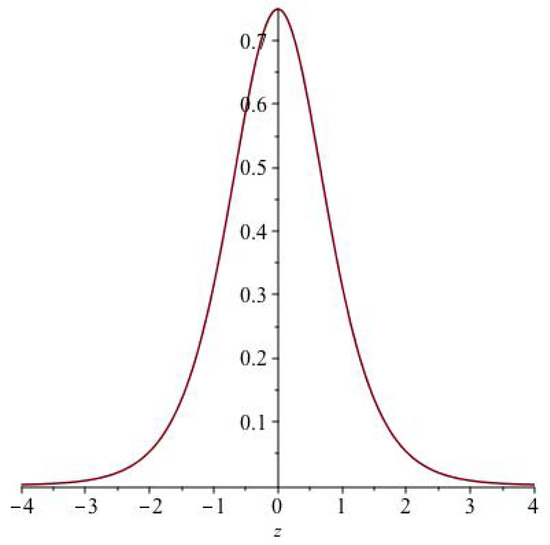Next Article in Journal
Securing Fingerprint Template Using Blockchain and Distributed Storage System
Next Article in Special Issue
Lie Symmetries and Low-Order Conservation Laws of a Family of Zakharov-Kuznetsov Equations in 2 + 1 Dimensions
Open AccessArticle

# Conservation Laws and Travelling Wave Solutions for Double Dispersion Equations in (1+1) and (2+1) Dimensions

byMaría Luz Gandarias 1,*,María Rosa Durán 1 andChaudry Masood Khalique 2,3,41
Departamento de Matemáticas, Universidad de Cádiz, P.O. Box 40, 11510 Puerto Real, Cádiz, Spain
2
International Institute for Symmetry Analysis and Mathematical Modelling, Department of Mathematical Sciences, North-West University, Mafikeng Campus, Private Bag X 2046, Mmabatho 2735, South Africa
3
College of Mathematics and Systems Science, Shandong University of Science and Technology, Qingdao 266590, Shandong, China
4
Department of Mathematics and Informatics, Azerbaijan University, Jeyhun Hajibeyli str., 71, AZ1007 Baku, Azerbaijan
*
Author to whom correspondence should be addressed.
Symmetry 2020, 12(6), 950; https://doi.org/10.3390/sym12060950
Received: 31 January 2020 / Revised: 16 March 2020 / Accepted: 18 March 2020 / Published: 4 June 2020
(This article belongs to the Special Issue Conservation Laws and Symmetries of Differential Equations)
In this article, we investigate two types of double dispersion equations in two different dimensions, which arise in several physical applications. Double dispersion equations are derived to describe long nonlinear wave evolution in a thin hyperelastic rod. Firstly, we obtain conservation laws for both these equations. To do this, we employ the multiplier method, which is an efficient method to derive conservation laws as it does not require the PDEs to admit a variational principle. Secondly, we obtain travelling waves and line travelling waves for these two equations. In this process, the conservation laws are used to obtain a triple reduction. Finally, a line soliton solution is found for the double dispersion equation in two dimensions. View Full-Text
Show FiguresFigure 1

MDPI and ACS Style

Gandarias, M.L.; Durán, M.R.; Khalique, C.M. Conservation Laws and Travelling Wave Solutions for Double Dispersion Equations in (1+1) and (2+1) Dimensions. Symmetry 2020, 12, 950.